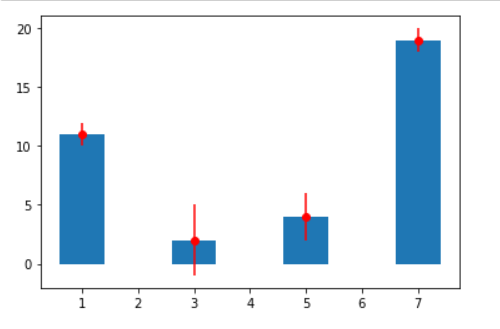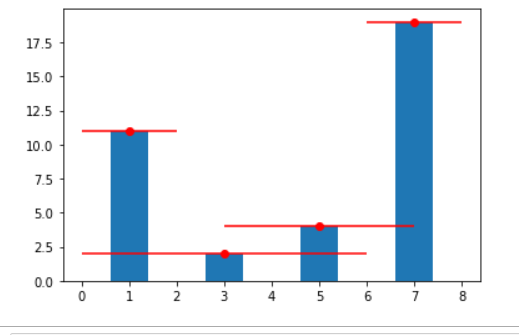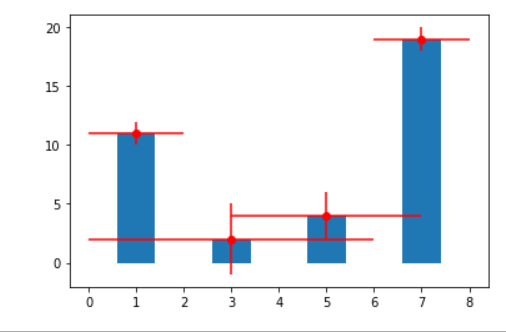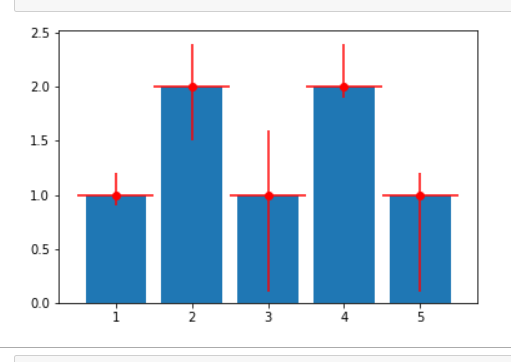Related Articles
Add error bars to a Matplotlib bar plot
• Last Updated : 17 Dec, 2020

Prerequisites: Matplotlib

In this article, we will create a bar plot with error bars using Matplotlib. Error bar charts are a great way to represent the variability in your data. It can be applied to graphs to provide an additional layer of detailed information on the presented data.

### Approach:

• Import required python library.
• Making simple data.
• Plot using plt.errorbar() function
• Display graph

The errorbar() function in pyplot module of matplotlib library is used to plot y versus x as lines and/or markers with attached errorbars.

Syntax: matplotlib.pyplot.errorbar(x, y, yerr=None, xerr=None, fmt=”, ecolor=None, elinewidth=None, capsize=None, barsabove=False, lolims=False, uplims=False, xlolims=False, xuplims=False, errorevery=1, capthick=None, \*, data=None, \*\*kwargs)

Parameters: This method accept the following parameters that are described below:

• x, y: These parameters are the horizontal and vertical coordinates of the data points.
• ecolor: This parameter is an optional parameter. And it is the color of the errorbar lines with default value NONE.
• elinewidth: This parameter is also an optional parameter. And it is the linewidth of the errorbar lines with default value NONE.
• capsize: This parameter is also an optional parameter. And it is the length of the error bar caps in points with default value NONE.
• barsabove: This parameter is also an optional parameter. It contains boolean value True for plotting errorsbars above the plot symbols.Its default value is False.

Implementation using the approach above is given below:

Example 1:  Adding Some errors in a ‘y’ value.

## Python3

 `# importing matplotlib``import` `matplotlib.pyplot as plt`` ` `# making a simple plot``a ``=` `[``1``, ``3``, ``5``, ``7``]``b ``=` `[``11``, ``2``, ``4``, ``19``]`` ` `# Plot scatter here``plt.bar(a, b)`` ` `c ``=` `[``1``, ``3``, ``2``, ``1``]`` ` `plt.errorbar(a, b, yerr``=``c, fmt``=``"o"``, color``=``"r"``)`` ` `plt.show()`

Output:Example 2:  Adding Some errors in the ‘x’ value.

## Python3

 `# importing matplotlib``import` `matplotlib.pyplot as plt`` ` `# making a simple plot``a ``=` `[``1``, ``3``, ``5``, ``7``]``b ``=` `[``11``, ``2``, ``4``, ``19``]`` ` `# Plot scatter here``plt.bar(a, b)`` ` `c ``=` `[``1``, ``3``, ``2``, ``1``]`` ` `plt.errorbar(a, b, xerr``=``c, fmt``=``"o"``, color``=``"r"``)`` ` `plt.show()`

Output:Example 3: Adding error in x and y

## Python3

 `import` `matplotlib.pyplot as plt`` ` `a ``=` `[``1``, ``3``, ``5``, ``7``]``b ``=` `[``11``, ``2``, ``4``, ``19``]`` ` `plt.bar(a, b)`` ` `c ``=` `[``1``, ``3``, ``2``, ``1``]``d ``=` `[``1``, ``3``, ``2``, ``1``]`` ` `plt.errorbar(a, b, xerr``=``c, yerr``=``d, fmt``=``"o"``, color``=``"r"``)``plt.show()`

Output:Example 4: Adding variable error in x and y.

## Python3

 `# importing matplotlib``import` `matplotlib.pyplot as plt`` ` `# making a simple plot``x ``=` `[``1``, ``2``, ``3``, ``4``, ``5``]``y ``=` `[``1``, ``2``, ``1``, ``2``, ``1``]`` ` `# creating error``y_errormin ``=` `[``0.1``, ``0.5``, ``0.9``, ``0.1``, ``0.9``]``y_errormax ``=` `[``0.2``, ``0.4``, ``0.6``,  ``0.4``, ``0.2``]`` ` `x_error ``=` `0.5`` ` `y_error ``=` `[y_errormin, y_errormax]`` ` `# ploting graph``plt.bar(x, y)`` ` `plt.errorbar(x, y,`` ` `             ``yerr``=``y_error,`` ` `             ``xerr``=``x_error,`` ` `             ``fmt``=``'o'``, color``=``"r"``)  ``# you can use color ="r" for red or skip to default as blue`` ` `plt.show()`

Output:Attention geek! Strengthen your foundations with the Python Programming Foundation Course and learn the basics.

To begin with, your interview preparations Enhance your Data Structures concepts with the Python DS Course.

My Personal Notes arrow_drop_up